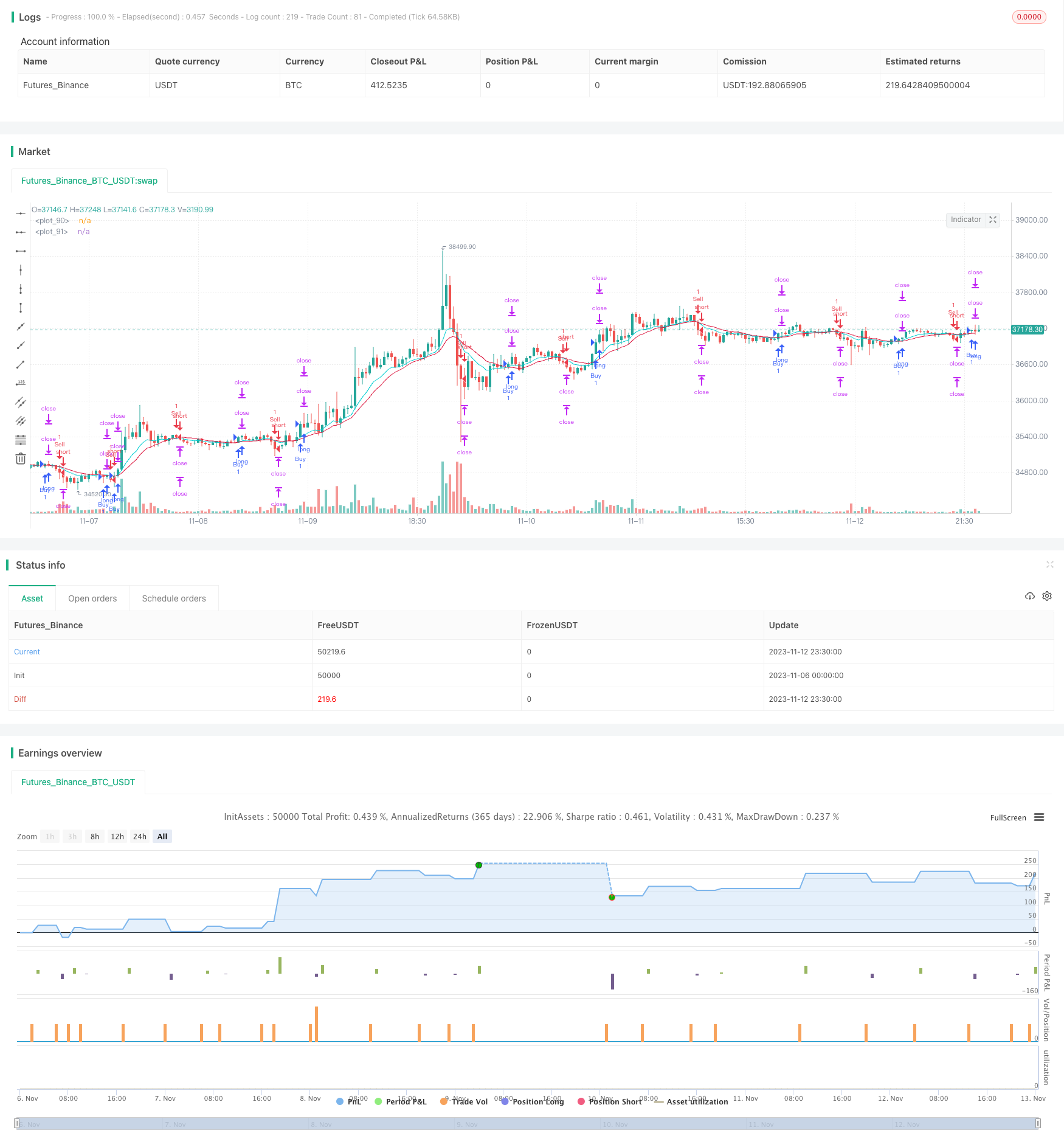# Dual Take Profit Moving Average Crossover Quantitative Strategy

Author: ChaoZhang, Date: 2023-11-14 16:04:33
Tags:### Overview

This strategy utilizes simple moving average crossover and dual take profit techniques to control risk and increase profitability. It is suitable for medium-term trading and capturing opportunities during trend changes.

### Strategy Logic

The strategy is based on EMA and WMA crossover to determine market trends. It goes long when EMA crosses above WMA, and goes short when EMA crosses below WMA.

Upon entry, two take profit levels are set. The first take profit is fixed at entry price + 20 pips, and the second take profit is fixed at entry price + 40 pips. Meanwhile, a stop loss is placed at entry price - 20 pips.

When price hits the first take profit, it will close out half of the position. The remaining position will keep running towards the second take profit or until stopped out.

There are three possible outcomes for each trade:

1. Price hits stop loss, takes 2% loss directly.

2. Price hits first take profit first, closes half position locking 1% profit, then keeps running until stopped out, ending with break even.

3. After hitting first take profit, price keeps running and hits second take profit, ending with 1% + 2% = 3% total profit.

The biggest advantage of this dual take profit strategy is that it controls risk and avoids huge single loss. Stop loss caps maximum loss within 2% when market moves against. The two take profits allow bigger gain when trend goes as expected.

Compared to single take profit/stop loss, this strategy has three outcomes - loss, win or break even, reducing the probability of stop loss. Even if stopped out, max loss is limited to 2%. Compared to traditional strategies, the dual take profit mechanism significantly reduces DD and improves win rate.

Another advantage is its simplicity. EMA and WMA are well-known indicators that are easy to understand. The take profit/stop loss logic is straightforward to monitor. These make the strategy easy to be adopted by beginners.

### Risk Analysis

Despite the advantages, there are also risks to be aware of for this strategy.

Firstly, as moving average indicators, EMA and WMA have relatively weak capabilities in identifying ranging market. Too many false signals may occur when trend is unclear, leading to over-trading.

Secondly, the fixed take profit/stop loss levels may not adapt to market volatility. They could be penetrated easily during high volatility, rendering them ineffective.

Lastly, the strategy cannot respond to unexpected events, with risk of being trapped. Major news events can create huge price gaps that directly breach the profit/loss levels, causing huge losses.

### Optimization Directions

There are several aspects to further optimize the strategy:

1. Improve entry signals. Test better moving average or trend indicators than EMA and WMA to generate higher quality signals.

2. Dynamically adjust take profit/stop loss. Use methods like ATR, trailing stop loss etc to make profit/loss levels adapt to markets.

3. Add filters. Require volume or secondary indicator confirmation before crossover to avoid traps. Also consider whether to trade around major events.

4. Optimize position sizing. Fine tune position size according to capital management rules.

### Conclusion

In summary, this is a simple and practical trend following strategy. It utilizes EMA and WMA crossover for entries, and dual take profit to control risks. Compared to traditional strategies, it has higher win rate and lower risk. Of course, limitations of the indicators and profit/loss settings should be watched out for. Further optimizations can make the strategy more robust.

```/*backtest
start: 2023-11-06 00:00:00
end: 2023-11-13 00:00:00
period: 30m
basePeriod: 15m
exchanges: [{"eid":"Futures_Binance","currency":"BTC_USDT"}]
*/

//@version=4
strategy("FS ATR & PS (MA)", overlay=true)

// Strategy
Sell = input(true)

// Time Period
start_year   = input(title='Start year'   ,defval=2019)
start_month  = input(title='Start month'  ,defval=1)
start_day    = input(title='Start day'    ,defval=1)
start_hour   = input(title='Start hour '  ,defval=0)
start_minute = input(title='Start minute' ,defval=0)
end_time     = input(title='set end time?',defval=false)
end_year     = input(title='end year'     ,defval=2019)
end_month    = input(title='end month'    ,defval=12)
end_day      = input(title='end day'      ,defval=31)
end_hour     = input(title='end hour'     ,defval=23)
end_minute   = input(title='end minute'   ,defval=59)

// MA
ema_period   = input(title='EMA period',defval=10)
wma_period   = input(title='WMA period',defval=20)
ema = ema(close,ema_period)
wma = wma(close,wma_period)

// Entry Condition
longCondition  =
nz(strategy.position_size) == 0 and
time > timestamp(start_year, start_month, start_day, start_hour, start_minute) and
(end_time?(time < timestamp(end_year, end_month, end_day, end_hour, end_minute)):true)

shortCondition =
crossunder(ema,wma) and Sell and
nz(strategy.position_size) == 0 and
time > timestamp(start_year, start_month, start_day, start_hour, start_minute) and
(end_time?(time < timestamp(end_year, end_month, end_day, end_hour, end_minute)):true)

// Exit Condition
a = input(20)*10
b = input(40)*10
c = a*syminfo.mintick
d = b*syminfo.mintick

long_stop_level     = float(na)
long_profit_level1  = float(na)
long_profit_level2  = float(na)
long_even_level     = float(na)

short_stop_level    = float(na)
short_profit_level1 = float(na)
short_profit_level2 = float(na)
short_even_level    = float(na)

long_stop_level     := longCondition  ? close - c : long_stop_level     
long_profit_level1  := longCondition  ? close + c : long_profit_level1  
long_profit_level2  := longCondition  ? close + d : long_profit_level2  
long_even_level     := longCondition  ? close + 0 : long_even_level     

short_stop_level    := shortCondition ? close + c : short_stop_level    
short_profit_level1 := shortCondition ? close - c : short_profit_level1 
short_profit_level2 := shortCondition ? close - d : short_profit_level2 
short_even_level    := shortCondition ? close + 0 : short_even_level    

// Position Sizing
Risk = input(defval=10, title="Risk per trade%", step=1, minval=0, maxval=100)/100
size  = 1

// Strategy
if longCondition
strategy.exit ("Exit1", stop=long_stop_level, limit=long_profit_level1, qty=size/2)
strategy.exit ("Exit2", stop=long_stop_level, limit=long_profit_level2)

if shortCondition
strategy.entry("Sell" , strategy.short, qty=size)
strategy.exit ("Exit3", stop=short_stop_level, limit=short_profit_level1, qty=size/2)
strategy.exit ("Exit4", stop=short_stop_level, limit=short_profit_level2)

// Plot
plot(strategy.position_size <= 0 ? na : long_stop_level    , color=#dc143c, style=plot.style_linebr, linewidth=1)
plot(strategy.position_size <= 0 ? na : long_profit_level1 , color=#00ced1, style=plot.style_linebr, linewidth=1)
plot(strategy.position_size <= 0 ? na : long_profit_level2 , color=#00ced1, style=plot.style_linebr, linewidth=1)
plot(strategy.position_size <= 0 ? na : long_even_level    , color=#ffffff, style=plot.style_linebr, linewidth=1)
plot(strategy.position_size >= 0 ? na : short_stop_level   , color=#dc143c, style=plot.style_linebr, linewidth=1)
plot(strategy.position_size >= 0 ? na : short_profit_level1, color=#00ced1, style=plot.style_linebr, linewidth=1)
plot(strategy.position_size >= 0 ? na : short_profit_level2, color=#00ced1, style=plot.style_linebr, linewidth=1)
plot(strategy.position_size >= 0 ? na : short_even_level   , color=#ffffff, style=plot.style_linebr, linewidth=1)
plot(ema,color=#00ced1)
plot(wma,color=#dc143c)

```

More